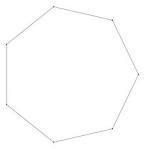Polygon and it's sidesA polygon has $n$ sides. Two of its exterior angles are 72 degrees and 35 degrees, while the remaining exterior angles are each equal to 23 degrees.

Find the value of $n$.

×